# Charles Plager's Latex Page

.

## Using Latex in PowerPoint (and Open Office)

There are several useful Latex packages out there for makins slides for presentations. But with more and more people using PowerPoint, it becomes hard to avoid. Fortunately, there are (at least) two very useful packages that let you embed Latex formulae in PowerPoint.

• TexPoint - Works to convert simple Latex commands into standard fonts, or used to embed complicated latex documents as bitmap images. Current version is now shareware, not freeware (only $25). • TeX4PPT - Requires PowerPoint 2002 or newer. Both of them require MikTeX or other version of Latex on Windows. See TeX4PPT's description of the difference If you are using OpenOffice, check out OOoLatex. I have not used it myself, but I hear good things about it. Note that if you can not/do not want to install these packages, you can use tex2png.pl below. ## tex2png.pl - Making PNG from Latex You can download the script tex2png.pl and run it from your computer (see the software requirements below). It is installed on the /cdf/home/ area on the CDF linux computers as /cdf/home/cplager/bin/tex2png.pl. This script not only makes a .png file, but also embeds the latex source code in the output file so images can easily be updated. For example (text I typed in brown): cplager@Fcdflnx4> ~cplager/bin/tex2png.pl -output broneside Please enter text, finished by <ctrl>-D on a blank line. {\mathcal B}(t\bar{t} \rightarrow W b Z c) = \frac{ N_\mathrm{candidates} - N_\mathrm{background} } {{\mathcal A} \cdot \int {\mathcal L} dt \cdot \sigma_{t \bar{t}}} Thank you. <img src="broneside.png" width="367" height="69">  This produces
Or even cplager@Fcdflnx4> tex2png.pl -output branch -white Please enter text, finished by <ctrl>-D on a blank line. {\mathcal B}(t \rightarrow Z c) = 0.5 \cdot ( 1 - \sqrt {1 - 2 \cdot {\mathcal B}(t\bar{t} \rightarrow W b Z c)} ) Thank you. <img src="branch.png" width="429" height="25">  produces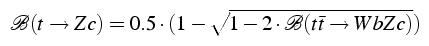You can even use color cplager@Fcdflnx4> tex2png.pl -output example Please enter text, finished by <ctrl>-D on a blank line. {\mathcal B}(t\bar{t} \rightarrow \colorbox{red}{\bf \color[rgb]{0,1,0} W b Z c}) = \frac{ N_\mathrm{candidates} - N_\mathrm{background} } {{\mathcal A} \cdot \int {\mathcal L} dt \cdot \sigma_{t \bar{t}}} Thank you. <img src="branch.png" width="449" height="71">  produces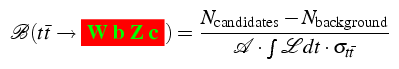You can give tex2png.pl a complete tex file as well. For the above example: cplager@Fcdflnx4> tex2png.pl -file complete.tex -output example  If you want to store just the latex snippet (i.e., latex source without the headers) in a file, you need to use < character: cplager@Fcdflnx4> tex2png.pl -o example < snippet.tex  To get a list of all options, use the -help option. cplager@Fcdflnx4> tex2png.pl -help usage: tex2png.pl [ options ] [texfile] Options: -debug Debug mode: doesn't delete temporary files -dpi n Set rendering dots per inch to n (default 150) -extract bla.png Extract latex source code from 'bla.png' -file Get input from file, not STDIN -gif Generate GIF image -help Print this message -output bla Outputs 'bla.png' -package XXX Includes package 'XXX' in header (can be used multiple times) -png Generate PNG image (default) -stdin Get tex content from STDIN (header and footer defined) -text Don't start in math mode with '-stdin' option -version Print version number -white Makes a white (instead of transparent) background tex2png.pl v1.6.1 Charles Plager cplager_tex2png[at]email(dot)plager[dot]net  ### Extracting Latex source from .png File To get the source code out of a png file that has been made with tex2png.pl, you use the script itself: cplager@Fcdflnx4> tex2png.pl -extract example.png Extracting tex source from example.png to example.tex  or cplager@Fcdflnx4> tex2png.pl -extract example.png savetexhere.tex Extracting tex source from example.png to savetexhere.tex  Note: Once the png file has been put into a Microsoft PowerPoint document, it looses the embedded latex source. ### History of tex2png.pl I modified textogif script from Fourmilab (isn't that cute) and ended up with tex2png.pl. I used png-tEXt.pl to learn how to embed comments in png files and ps2gif.sh to learn how to go from postscript files to png and gif files (I recommend my perl version ps2png.pl if you want to embed the converting functions in another perl script). ### Requirements for tex2png.pl In order to run this on your computer, you need: • Perl version ≥ 5.6 • Perl library Compress::Zlib (usually installed by default) • LaTeX 2e • dvips • Netpbm If you are getting Perl errors on the fcdflnxN machines, try running setup perl v5_8 before running the script. ## Latex Snippets This is a random (and growing) collection of formulas and techniques I find useful. If you have any suggestions, please let me know. Latex codeOutput • (no options needed) \mathrm{Transverse~Mass} = \sqrt { (\sum E_T)^2 {\color{red} -} (\sum \vec{p_T})^2} •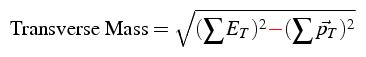• (Uses -text option) \begin{tabular}{r r} Longitudinal &$\frac{3}{4} (1 - cos^2(\theta^*))$\\ & \\ Left Handed &$\frac{3}{8} (1 - cos(\theta^*))^2$\\ & \\ Right Handed &$\frac{3}{8} (1 + cos(\theta^*))^2$\\ \end{tabular} •
• (Uses -text option) $\delimiterfactor=1200 % 1.2 x bigger brace y = \left\{% \begin{array}{ll} x^2+2x &\textrm{if }x<0,\\ x^3 &\textrm{if }0\le x<1,\\ x^2+x &\textrm{if }1\le x<2,\\ x^3-x^2 &\textrm{if }2\le x. \end{array}% \right.$  •
• (Uses -text -package colortbl options) Color by column:\\\vskip 2mm \begin{tabular} {|>{\columncolor{cyan}}l| >{\color{red} \columncolor{green}}c| >{\columncolor{yellow}}r|} \hline one & two & three\\ one & two & three\\ one & two & three\\ \hline \end{tabular}  •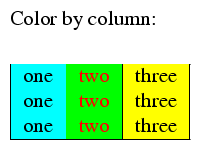• (no options needed) \overbrace{a+\underbrace{b+c}_\textrm{Something Under}+d}^\textrm{Something Over}  •
• (Uses -text option) \def\met{\ensuremath{\not\!\!{E_{T}}}} {\Huge \met}, {\large \met}, {\small \met} and$\met\$.

•
• (Uses -text option)
\begin{eqnarray*}
% First Line
\textrm{Expected Limit of }(\mathrm{Mass}^2) &=&
\sum P(i | n_b) \cdot (FC_\mathrm{mass} (i | A, n_b))^2 \\
% Second Line
\textrm{Expected Limit of Mass}~~~~~ &=&
\sum P(i | n_b) \cdot ~\,FC_\mathrm{mass} (i | A, n_b) \\
% Third Line
\textrm{Expected Limit of }(\mathrm{Mass}^2) &\neq&
(\textrm{Expected Limit of Mass})^2
\end{eqnarray*}

•